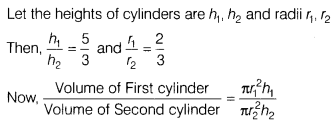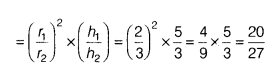# The heights of two cylinder are in the ratio 5:3 and their radii are in the ratio 2:3. Then, find the ratio of their volumes

The heights of two cylinder are in the ratio 5:3 and their radii are in the ratio 2:3. Then, find the ratio of their volumes.Hence, required ratio of volumes of cylinders is 20:27.